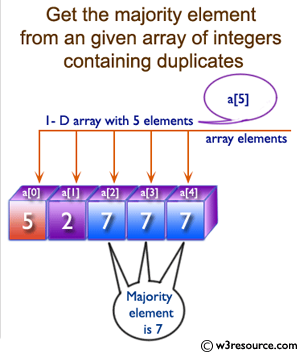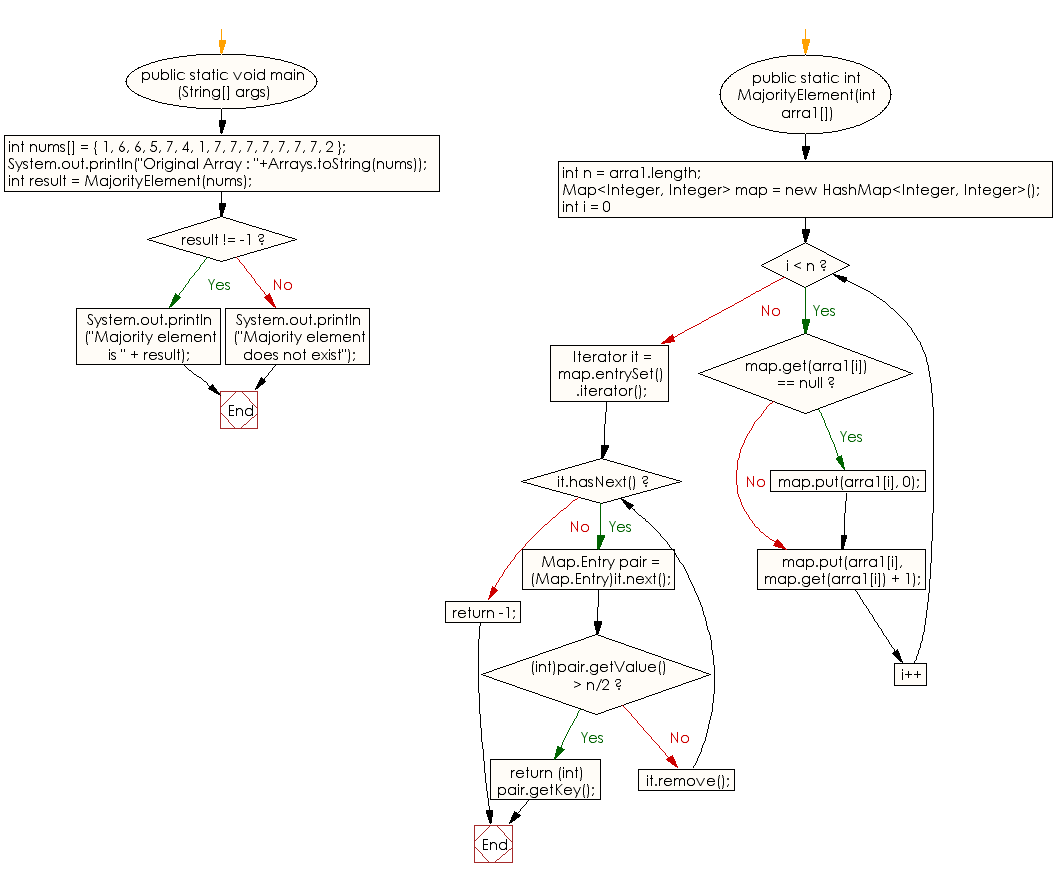﻿ Java: Find majority element in an array contains duplicates# Java Array Exercises: Get the majority element from a given array of integers containing duplicates

## Java Array: Exercise-38 with Solution

Write a Java program to get the majority element from a given array of integers containing duplicates.

Majority element: A majority element is an element that appears more than n/2 times where n is the size of the array.

Pictorial Presentation:Sample Solution:

Java Code:

``````import java.util.HashMap;
import java.util.Map;
import java.util.Iterator;
import java.util.Arrays;

public class Main
{
public static void main (String[] args)
{
// Array - test majority element
int nums[] = { 1, 6, 6, 5, 7, 4, 1, 7, 7, 7, 7, 7, 7, 7, 2 };
System.out.println("Original Array : "+Arrays.toString(nums));
int result = MajorityElement(nums);
if (result != -1)
System.out.println("Majority element is " + result);
else
System.out.println("Majority element does not exist");
}

public static int MajorityElement(int arra1[])
{
int n = arra1.length;

// Hash Map
Map<Integer, Integer> map = new HashMap<Integer, Integer>();

// Element's frequency in a map
for (int i = 0; i < n; i++)
{
if (map.get(arra1[i]) == null)
map.put(arra1[i], 0);

map.put(arra1[i], map.get(arra1[i]) + 1);
}

// Return the element if its count is more than n/2
Iterator it = map.entrySet().iterator();
while (it.hasNext())
{
Map.Entry pair = (Map.Entry)it.next();
if ((int)pair.getValue() > n/2)
return (int)pair.getKey();

it.remove();
}

// no majority element
return -1;
}
}
```
```

Sample Output:

```
Original Array : [1, 6, 6, 5, 7, 4, 1, 7, 7, 7, 7, 7, 7, 7, 2]
Majority element is 7
```

Flowchart:Visualize Java code execution (Python Tutor):

Java Code Editor:

Improve this sample solution and post your code through Disqus

What is the difficulty level of this exercise?

Test your Programming skills with w3resource's quiz.

﻿

## Java: Tips of the Day

countOccurrences

Counts the occurrences of a value in an array.

Use Arrays.stream().filter().count() to count total number of values that equals the specified value.

```public static long countOccurrences(int[] numbers, int value) {
return Arrays.stream(numbers)
.filter(number -> number == value)
.count();
}
```

Ref: https://bit.ly/3kCAgLb